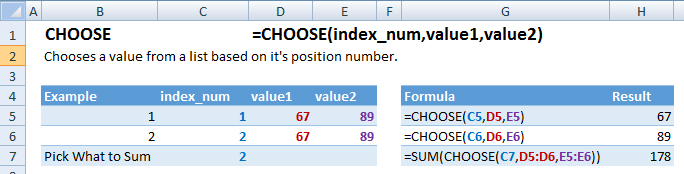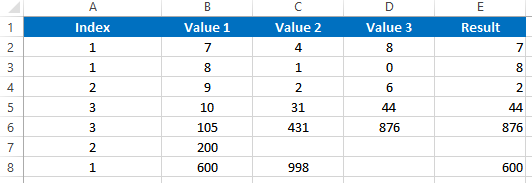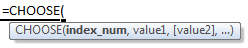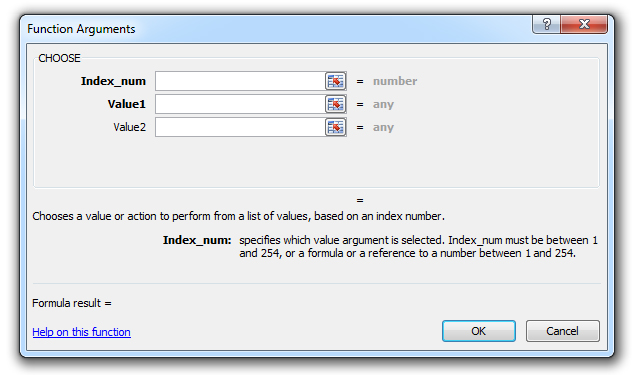# CHOOSE Function – Examples in Excel, VBA, Google Sheets

This Excel Tutorial demonstrates how to use the Excel CHOOSE Function in Excel to choose a value from a list, with formula examples.

## CHOOSE Function Description:

The CHOOSE Function Chooses a value from a list based on it’s position number.

## Formula Examples:Example Formula Result
1 =CHOOSE(C5,D5,E5) 67
2 =CHOOSE(C6,D6,E6) 89
Pick What to Sum =SUM(CHOOSE(C7,D5:D6,E5:E6)) 178

## Syntax and Arguments:

The Syntax for the CHOOSE Formula is:

`=CHOOSE(index_num,value1,value2)`

### Function Arguments ( Inputs ):

index_num – A number indicating which value to choose.

value1 – The list from which to select. You may create a list within the function input, reference a list in a worksheet, or reference a named range. Your list may include cell references, text, functions, formulas, or values.

value2

Use the CHOOSE Function to choose a value or a calculation from a list based on it’s position number.

## CHOOSE Examples in VBA

You can also use the CHOOSE function in VBA. Type:
`application.worksheetfunction.choose(index_num,value1,value2)`

Executing the following VBA statements

```Range("E2")=Application.WorksheetFunction.Choose(Range("A2"),Range("B2"),Range("C2"),Range("D2"))
Range("E3")=Application.WorksheetFunction.Choose(Range("A3"),Range("B3"),Range("C3"),Range("D3"))
Range("E4")=Application.WorksheetFunction.Choose(Range("A4"),Range("B4"),Range("C4"),Range("D4"))
Range("E5")=Application.WorksheetFunction.Choose(Range("A5"),Range("B5"),Range("C5"),Range("D5"))
Range("E6")=Application.WorksheetFunction.Choose(Range("A6"),Range("B6"),Range("C6"),Range("D6"))
Range("E7")=Application.WorksheetFunction.Choose(Range("A7"),Range("B7"),Range("C7"),Range("D7"))
Range("E8")=Application.WorksheetFunction.Choose(Range("A8"),Range("B8"),Range("C8"),Range("D8"))```

will produce the following resultsFor the function arguments (index_num, etc.), you can either enter them directly into the function, or define variables to use instead.

`=AND(`